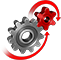# WolframSystemModeler

## Modeling the World

The world model is one of the most famous applications of the system dynamics methodology, here implemented using the System Dynamics library.

### Model OverviewModeling the world.
The top-level model diagram contains 13 submodels ranging from human fertility to pollution dynamics, to mention a few. In total, this model contains around 300 variables.

### Predicting the Future of the World

Here we compare the original estimates of population growth from the years 1900 to 2100 with how it could be if legislators enforced some major policy changes in terms of: (1) efficiency; (2) industrial output; and (3) a maximum of two children per family. We’ll also see what the effect would be if these policies were introduced in either 1982 or in 2012.In the left panel, validation data from Wolfram|Alpha has been overlaid on the simulations. The date of the last model update has also been highlighted. As can be seen, the model predicts a rather drastic decline in life expectancy and total population if the world carries on as usual. Enforcing the policy changes would result in an increase in overall life expectancy, and eventually the population curve would reach a more stable state at a level where our natural resources could support us.
Judging from the simulation results, it seems that these policy changes would have a significant impact on both population growth and life expectancy. But would any of these changes have a long‐term effect? Twenty-five generations from now, would the natural resources have become depleted?As can be seen here, the world population does decrease in the two other cases as well. This was to be expected, however, since one of the major policy changes meant only allowing two children per family.
This is a model, and since a model is by definition an approximation of a complex process, it of course has its limitations (e.g. the model slightly overestimates the population growth, as can be seen if one compares with the Wolfram|Alpha data). These scenarios assume that nothing else changes and cannot for instance predict the impact of novel technologies that could reduce our need for natural resources.

Integrate Wolfram|Alpha with your modeling process

Validate your model with data from Wolfram|Alpha.

## Wolfram System Modeler

Questions? Comments? Contact a Wolfram expert »